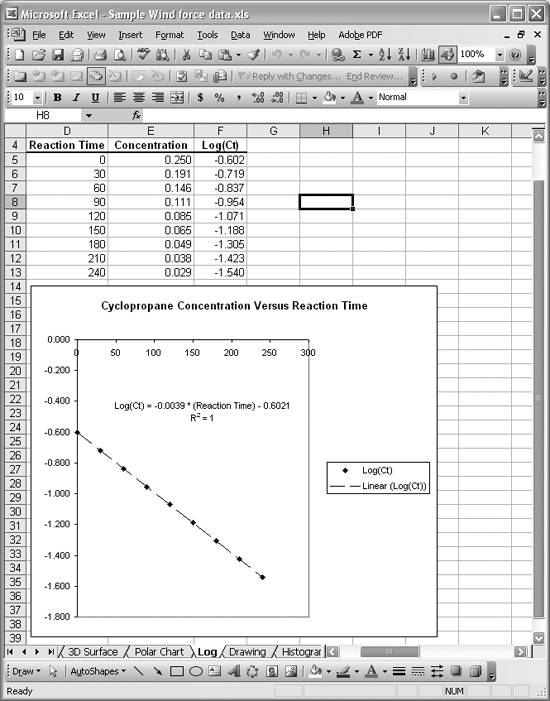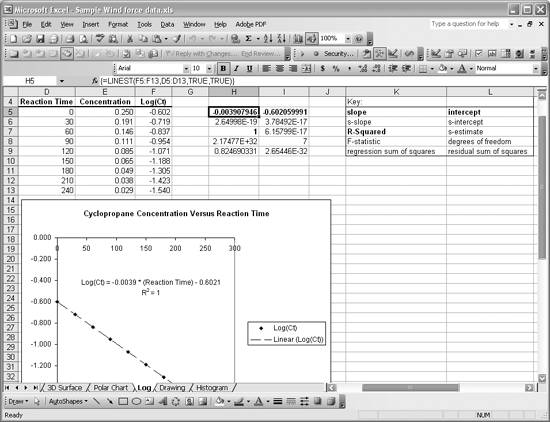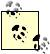# Using a Single Spreadsheet Function for Linear Curve Fitting

Problem

You want to perform a quick linear curve fit without using chart trendlines and without having to write the least-squares formulas yourself.

Solution

Use Excel's LINEST function.

Discussion

LINEST computes statistics for a least-squares straight line through a given set of data. The syntax for LINEST is {= LINEST(y-value cell range, x-value cell range, compute intercept, compute statistics)}. Note the braces surrounding this formula since it is an array formula. When you type this formula into a cell, you have to press Ctrl-Shift-Enter to enter it. Further, you have to select a 2 x 5 grid of cells before typing and entering the formula. This is because LINEST returns an array of data containing the various statistics computed for the best-fit line.

The first argument in LINEST is a cell range containing the y-values for the data to be fit, and the second argument is a cell range containing the x-values. The third argument is a logical value (true or false) specifying whether or not to force the intercept of the fit line to pass through zero. If TRue, the intercept is calculated in the usual least-squares manner. If false, the intercept is forced to zero, with the slope computed accordingly. The fourth argument is a logical value indicating whether or not to display extended statistics for the best-fit line. These extended statistics include such things as standard errors and residual sums. (See the "LINEST" help topic in Excel's online help for more information on these statistics. Also, take a look at Recipes 8.7 and 8.8.)

Consider the chemical reaction data discussed in Recipe 4.5. Instead of plotting concentration versus reaction time on a semilog scale, you can plot the log of the concentration versus reaction time on a linear scale. The plot should look like a straight line. Figure 8-6 shows the log of the concentration versus reaction time data, along with an XY scatter plot of the data using linear axes.

Figure 8-6. Log concentration versus reaction timeI also included a linear trendline on this chart (see Recipe 8.1). The linear trendline represents the data very well.

Figure 8-7 shows the results of using LINEST to perform a linear fit. The data returned by LINEST is contained in cells H5 to I9. The key to the right describes what each returned value represents. The three values in bold type are the slope, intercept, and R-squared value. These values agree very well with those returned by the chart trendline.

Figure 8-7. Linear fit using LINESTYou'll also notice that I have cell H5 selected. This is the first cell in the range that I selected before entering the formula. The formula I entered is displayed in the formula bar. The actual formula is =LINEST(F5:F13,D5:D13,TRUE,TRUE). The y-values are contained in the range F5:F13. These are the log of concentration values. The x-values are the reaction times in the range D5:D13. I set the final two arguments to trUE so that LINEST computes the intercept and returns extended statistics.

Here are the steps for using LINEST as I did in this example:

1. With the mouse, select the cell range H5:I9. (You can select any 2 x 5 range you'd like). If you want to see only the slope and intercept, select only two side-by-side cells.
2. Type the LINEST formula using the syntax described earlier.
3. Don't press Enter! Press Ctrl-Shift-Enter to enter the formula as an array formula.

That's all there is to it.Excel also includes a function called LOGEST that performs an exponential fit. It's used in much the same way as LINEST. See the Excel help topic "LogEst" for more information.

LINEST is also capable of performing multiple linear regression where the equation fit is of the form y = m1x1 + m2x2 + m3x3 + ... + mixi + b. See Recipe 8.4 for an example.The Analysis ToolPak add-in contains a regression tool that is capable of performing linear and multiple linear regressions. This tool actually uses LINEST to compute the curve fits, and it also returns a boatload of other statistics including ANOVA (analysis of variance) results and confidence intervals. It also computes residuals, making it convenient to check them while assessing the fit. It also prepares a few plots for you. The Analysis ToolPak is installed on your version of Excel if you see the menu item Data Analysis under the Tools menu. Otherwise, select Tools [Image_Link]/images/U2192.jpg border=0> Add-Ins...from the main menu bar and select Analysis ToolPak from the list of available add-ins.

Read the help topic "LinEst" in Excel's online help guide for more details on the LINEST function.Excel Scientific and Engineering Cookbook (Cookbooks (OReilly))
ISBN: 0596008791
EAN: 2147483647
Year: N/A
Pages: 206
Authors: David M Bourg